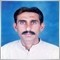# Adaptive lookback indicators - page 107162736

krelian99:
I am having a problem with this : smi has 3 periods and if we make all the 3 periods vhf adaptive, the results is unpredictive in some case. Trying to find some solution for that, but so far no acceptable results1202

I am having a problem with this : smi has 3 periods and if we make all the 3 periods vhf adaptive, the results is unpredictive in some case. Trying to find some solution for that, but so far no acceptable results

We have Length, Smooth 1 and Smooth 2. At phase accumulation adaption we adapted the length only. Is it here not possible?

double alfa1 = 2.0 / (1.0 + Smooth1);
double alfa2 = 2.0 / (1.0 + Smooth2);

for (i=limit, r=Bars-i-1; i>=0; i--,r++)
{
double Length = iHilbertPhase(wrkBuffer[r],PaFilter,PaCycles,i);
double highest = High[iHighest(NULL,0,MODE_HIGH,Length,i)];//SMIPeriod1
double lowest  = Low [iLowest (NULL,0,MODE_LOW ,Length,i)];//SMIPeriod1
double sm      = Close[i]-(highest+lowest)*0.5;
double hl      =           highest-lowest;

The two smoothers I have to optimize. Is extra double smoothing option still reasonable here?162736

krelian99:

We have Length, Smooth 1 and Smooth 2. At phase accumulation adaption we adapted the length only. Is it here not possible?

double alfa1 = 2.0 / (1.0 + Smooth1);
double alfa2 = 2.0 / (1.0 + Smooth2);

for (i=limit, r=Bars-i-1; i>=0; i--,r++)
{
double Length = iHilbertPhase(wrkBuffer[r],PaFilter,PaCycles,i);
double highest = High[iHighest(NULL,0,MODE_HIGH,Length,i)];//SMIPeriod1
double lowest  = Low [iLowest (NULL,0,MODE_LOW ,Length,i)];//SMIPeriod1
double sm      = Close[i]-(highest+lowest)*0.5;
double hl      =           highest-lowest;

The two smoothers I have to optimize. Is extra double smoothing option still reasonable here?

There is length (that is the calculation length that is used to calculate the SMI range) and the two periods that are used for smoothing (since SMI is a sort of a double smoothed indicator). First period (the "length") is hard to adapt, since it must be bar bound - it must be an integer). The second two can be adapted, but having two different periods for smoothing, making them adaptive depending on their original lengths can cause "period inversion" - in some cases what was longer can become shorter than the other period, and vice versa. That is the sort of the problems I am talking about this time162736

krelian99:

We have Length, Smooth 1 and Smooth 2. At phase accumulation adaption we adapted the length only. Is it here not possible?

double alfa1 = 2.0 / (1.0 + Smooth1);
double alfa2 = 2.0 / (1.0 + Smooth2);

for (i=limit, r=Bars-i-1; i>=0; i--,r++)
{
double Length = iHilbertPhase(wrkBuffer[r],PaFilter,PaCycles,i);
double highest = High[iHighest(NULL,0,MODE_HIGH,Length,i)];//SMIPeriod1
double lowest  = Low [iLowest (NULL,0,MODE_LOW ,Length,i)];//SMIPeriod1
double sm      = Close[i]-(highest+lowest)*0.5;
double hl      =           highest-lowest;

The two smoothers I have to optimize. Is extra double smoothing option still reasonable here?

Here is a kind of a compromise : adaptive is just the longer smi calculation period. Even like that the difference is sometimes significant. Try it out

PS: newest set of averages included too

Files:1202

Here is a kind of a compromise : adaptive is just the longer smi calculation period. Even like that the difference is sometimes significant. Try it out

PS: newest set of averages included too

Nice and smooth, I'll test it. Thank you so much, mladen.24

Here is a kind of a compromise : adaptive is just the longer smi calculation period. Even like that the difference is sometimes significant. Try it out

PS: newest set of averages included too

thanks, great job again Mladen !

will it be possible to do the same thing with blau-Tsi  (blau tsi averages) ?162736

siegfriednovell:

thanks, great job again Mladen !

will it be possible to do the same thing with blau-Tsi  (blau tsi averages) ?

Will check that too :)4557

Here is a kind of a compromise : adaptive is just the longer smi calculation period. Even like that the difference is sometimes significant. Try it out

PS: newest set of averages included too

Thanks so much for adding adaptive and newest averages and Yes the difference is not too much as you was telling already,also where some times significant there also increased numbers of signals :)

regards676

mntiwana:

Thanks so much for adding adaptive and newest averages and Yes the difference is not too much as you was telling already,also where some times significant there also increased numbers of signals :)

regards

What on lower time frames (with bigger volatility in data)?4557

sebastianK:
What on lower time frames (with bigger volatility in data)?

I think did not get your question,can you explain a bit more.

if you asking about indicator used in the picture it is "blau - smi averages 2.2"

newest one from the post number 1065 at previous page on this same thread.